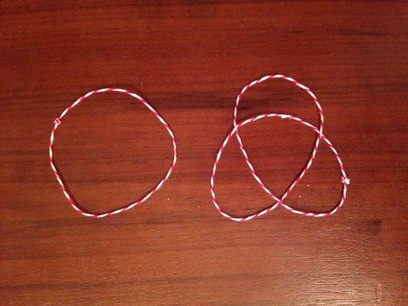mrb : home

# #

### Knots and a Mathematical Notion of Equivalence

A knot, like everything else in mathematics, has a very specific definition. Let’s look at a photo:Left: An unknot, Right: A trefoil

Both of these are the kinds of knots that mathematicians interested in knots study: they are joined at the end. The unknot above is a trivial knot, the simplest kind of knot imaginable. The knot on the right is a trefoil, a simple sort of knot that is not equivalent to an unknot.

Practical and theoretical computer science are both concerned with notions of equivalence. Simple values are easy to equate in almost any system, while the ability of a programming language to allow the programmer to reason about equality in simple but expressive ways is highly valued.

What do we mean when we consider equality of abstract types?

If you take the time to create the two knots above, you can get a physical sense for how they are not equivalent in the knot theory sense. This level of intuitive connection to foundational concepts of our theoretical frameworks is what we should strive for in our models as practical and theoretical computer scientists.

#### Works Cited

 http://en.wikipedia.org/wiki/Knot_theory

 “Terese Lite,” Excerpts from the book TERM REWRITING SYSTEMS by Terese. From the Course Term Rewriting Systems at the Vrije Universiteit, fall 2006.